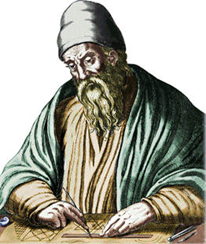# Primal Soup

Posted by Graham Wheeler on Wednesday, January 6, 2010Number theory really began with Euclid, around 300BC, in books 7 through 9 of his masterwork, The Elements. It is here that we find the original definitions of odd and even numbers, prime and composite numbers, perfect numbers (numbers which are the sum of their factors, e.g. 6 = 3 + 2 + 1), and more. But the greatest achievements of all were his proofs that composite numbers are the products of primes, that this factorization is unique, and that there are an infinity of primes. Most introductory algebra or number theory classes cover these three great proofs, but they are worth revisiting for those who may have forgotten.

Before doing so, it is worth revisiting Euclid’s clever algorithm for calculating the greatest common divisor (GCD) of two numbers. We made use of this when solving the Monkey and Coconuts puzzle.

We divide the smaller number into the larger and keep track of the remainder. Then we divide that remainder into the smaller number to get a second remainder, then divide the second remainder into the first remainder to get a third remainder, and so on. Eventually we get a remainder of zero; the remainder just before this is the GCD. If this happens to be one, we say the numbers are coprime. For example, consider 64 and 10:

• 64 = 10 x 6 + 4
• 10 = 4 x 2 + 2
• 4 = 2 x 2 + 0

So the GCD is 2.

For another example, consider 77 and 65:

• 77 = 65 x 1 + 12
• 65 = 12 x 5 + 5
• 12 = 5 x 2 + 2
• 5 = 2 x 2 + 1
• 2 = 1 x 2 + 0

So the GCD is 1 and the numbers are coprime.

In the proofs below we make use of a special case of Bézout’s identity, which states that if two numbers $$a$$ and $$b$$ have a GCD $$g$$, then there exists some $$x$$ and $$y$$ such that $$ax + by = g$$. In our case we care about the situation where $$a$$ and $$b$$ are coprime, so that there exists some $$x$$ and $$y$$ such that $$ax + by = 1$$. We aren’t proving that here but it is exactly equivalent to solving the Diophantine equations we solved for the Monkey and Coconuts problem (where $$x$$ and $$y$$ where the number of coconuts at the start and end).

Proof that Composite Numbers have Prime Factors

Let $$A$$ be a composite number. Then by definition $$A$$ must be divisible by some smaller number $$B$$. If $$B$$ is prime we are done; if $$B$$ is not prime it is must be divisible by some smaller number $$C$$, and so on. Continuing in this way, we find:

$$A > B > C > \dots > 1$$

This sequence must terminate with a prime number in the last position before 1; if not, that is, if each successive number is still composite, then the series is infinite, but we cannot have an infinite series of decreasing whole numbers.

Prime Factorization Theorem (aka the Unique Factorization Theorem aka the Fundamental Theorem of Arithmetic)

There are two steps: first we show that if a prime $$p$$ divides a composite $$ab$$, then $$p$$ must divide at least one of $$a$$ or $$b$$. Assume $$p$$ does not divide $$a$$ - then the GCD (greatest common divisor) of $$p$$ and $$a$$ must be 1, as $$p$$ is prime. By Bézout’s identity, there must be some integers $$x$$ and $$y$$ satisfying $$px + ay = 1$$. Multiplying both sides by $$b$$ gives $$pxb+aby = b$$, and both terms on the left hand side are products of $$p$$, thus $$b$$ must be a product of $$p$$.

Now for the main proof. Let $$s$$ be the smallest natural number that can be written as a product of prime numbers in more than one way (ignoring ordering, of course). Let one factorization be $$p_1 p_2 \dots p_m$$ and another be $$q_1 q_2 \dots q_n$$. The previous result proves that $$p_1$$ divides either $$q_1$$ or $$q_2 \dots q_n$$. Because both $$q_1$$ and $$q_2 \dots q_n$$ must have unique prime factorizations, $$p_1$$ must equal some $$q_i$$. If we then remove $$p_1$$ and $$q_i$$  we have two different factorizations of a number $$\frac{s}{p_1}$$ which is smaller than $$s$$, which contradicts our assumption that $$s$$ is the smallest such number.

Proof of the Infinitude of the Primes

Assume the set of primes is finite, given by $${ p_1, p_2, \dots, p_n }$$. Consider the number $$p = p_1 p_2 p_3 \dots p_n + 1$$. This is not divisible by any of the primes in the set; it always leaves a remainder of 1. Therefore it is either itself prime or has other prime divisors not in the set, and so the set is incomplete.

Further reading: William Dunham’s Journey through Genius: The Great Theorems of Mathematics has two chapters on Euclid’s elements and covers much of this material (although he omits the proofs apart from the last one). Proofs from THE BOOK has about six different proofs of the infinitude of the primes, each using a different branch of mathematics, from algebra to set theory to topology. To dust off my rusty memory of these proofs I had to pull out my well-thumbed college textbook Rings, Fields and Groups: An Introduction to Abstract Algebra, but while it is a great text I wouldn’t recommend it to those faint of heart!